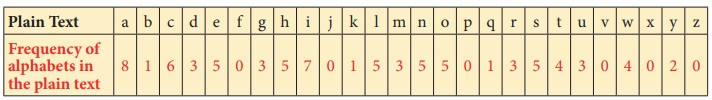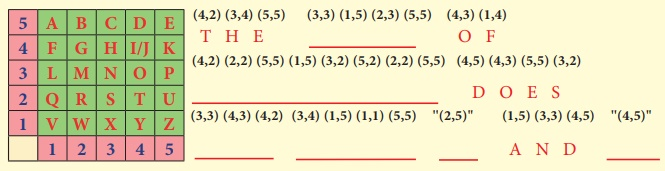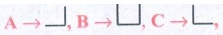Home | | Maths 8th Std | Cryptology

# Cryptology

1. Cryptology – Some technical details 2. Examples of Cipher Code

Cryptology

In today’s world, security in information is a fundamental necessity not only for military and political departments but also for private communication. Today’s world of communication has increased the importance of financial data exchange, image processing, biometrics and e-commerce transaction which in turn has made data security an important issue. Cryptology is defined as the science which is concerned with communication in secured form.

1. Cryptology – Some technical details

Plain text: The original message is called plain text.

Cipher text or Cipher number: The encrypted output (converted message into code) is called Cipher text or Cipher number. Cipher text is written in capital letters, while plain text is usually written in lowercase. A secret key is to use something to generate the Cipher text from the plain text.

Encryption and Decryption: The process of converting the plain text to the Cipher text is called encryption and the vice versa is called decryption.

Let us try to create some Cipher text that we use in the form of coded message at some point in our real life.

2. Examples of Cipher Code

1. Shifting Cypher Text

Ceasar Cipher

The Ceasar Cipher is one of the earliest known and simplest ciphers. It is a type of substitution cipher in which each letter in the text is “shifted” a certain number of places down the alphabets.

To pass an encrypted message from one person to another, it is first necessary that both parties have the ‘key’ for the cipher, so that the sender may encrypt it and the receiver may decrypt it. For the Caesar Cipher, the “key” is the number of characters to shift the cipher alphabet. So, we have to know how big the switch is to break the code.

Let us know about more Ciphers from the following examples and situations.

Example 7.8

Use Ceasar Cipher table set +4 and try to solve the given secret sentence.

fvieo mr gshiw ger fi xvmgoc

Solution:

Let us make Ceasar Cipher table first. Here, we have to set to +4 table.

For that, we have to start letter e to set as A, f as B … likewise d as Z.

Now, the +4 Ceasar Cipher table looks likeThe given plain text is

fvieo mr gshiw ger fi xvmgoc

To crack this secret code, follow the steps given below.

Step 1: Using Ceasar Cipher table, let us first match the most repeated letters. This will help us to progress faster.

fvieo mr gshiw ger fi xvmgocStep 2: Then, let us find remaining letters to complete the code.Thus, the secret sentence is decoded as, BREAK IN CODES CAN BE TRICKY

2. Substituting Cypher Text

Each letter in a text is “substituted” by certain pictures and symbols, a key message, group of words, letters or a combination of these can be used to encode or decode the information. Let us learn more from the following situation.

Situation:

The teacher divides the class into two teams and displays a worksheet given in the figure below for students to complete. Play a game, teams take turns suggesting letter substitutions and someone entering your suggestions in the table. Teams earn points for correct letter guesses.

Worksheet 1 :- Additive Cipher [key = 5]

Convert a given plain text into Additive Cipher text (number) code.

“ mathematics is a unique symbolic language in which the whole world works and acts accordingly.”

• Especially for additive Ciphers, you only need key number. You can complete the cipher table by filling in the rest of the numbers in order.

• If you can, find and make frequency table for alphabets, it is help you to get started.

• Match the most repeated letters first and fill the Cipher number.

• Look for familiar one-letter words like a or i. common two and three letter words like of, to, in, it, at, the, and, for, you …..

• Look for consecutive numbers in the Cipher text and match them with possible consecutive letters in the plain text.

Now, to start converting a plain text into Cipher number, first we have to make a cipher table as shown below. Here there key is 5. As per key number, we have to start and fill a = 05, b = 06 …….. z = 04 respectively.To start encoding the text, let us count the frequency of alphabets and frame frequency table as shown below.With the help of Cipher table and frequency table, let us fill the Cipher numbers for the plain text. Let us first match the most repeated letters (5 times and above), then 2 to 4 times repeated letters and finally remaining letters step by step as shown in given figure.Thus, the Additive Cipher text for plain text is as follows:

“17 05 24 12 09 17 05 24 13 07 23 13 23 05 25 18 13 21 25 09 23 03 17 06 19 16 13 07

16 05 18 11 25 05 11 09 13 08 01 12 13 07 12 24 12 09 01 12 19 16 09 01 19 22 16 08

01 19 22 15 23 05 18 08 05 07 24 23 05 07 07 19 22 08 13 18 11 16 03”

Activity

TREASURE HUNT

Treasure in the Mathematics Club room

The teacher divides the students into four groups and gives each group a code and a clue and then asks them to cracking the clues to find

(i) the identity of the treasure

(ii) the place of the treasure

(iii) the room in which the treasure is present.

You may take notes on this piece of paper as you proceed through the search

Code 1: Pigpen

Message:- If you decode the clue here you can get the four expected treasure names

I. Fill in the blank boxes and decode

The Pigpen code looks like meaningless writing, but it is quite easy to catch on to.

Each letter is represented by the part of the “Pigpen” that surrounds it.

The first code uses the following key. To complete the code, you need to work out how to use the key to decode the message.Code 2: Polybius Square Cipher

Message:- If you decode this you can get the clue to identify the name of the treasure name

II. Fill in the blanks

A Polybius Square is a table that allows someone to convert letters into numbers.

Use the Polybius square rows and column values to find the codeUsing this a set of numbers can be used to denote a letter and vise versa

(4, 2) represent T, (3, 4) represent H, (5, 5) represent E Likewise, when we solve for the remaining, we get

THE NAME OF TREASURE DOES NOT HAVE B AND D.

Since clue 2 gives that the treasure item does not have the letters b & d, therefore "exam pad" & "geometry box" cannot be the treasure item.

Hence, the treasure item is "gift voucher"

Code 3: Atbash Cipher

Message:- If you decode this you can get the clue room number where the treasure is present

(It may be 24 or 25 or 26 or 27 or 28 or 29 or 30)

III. Find the code using the key as shown in given figure:

Atbash cipher is a substitution cipher with just one specific key where all the letters are reversed that is A to Z and Z to A.GSV ILLN MFNYVI RH Z NFOGRKOV LU ULFI ZMW HVEVM

The given Cipher is:

GSV ILLN MFNYVI RH Z NFOGRKOV LU ULFI ZMW HVEVM

Decoded Message :

THE ROOM NUMBER IS A MULTIPLE OF FOUR AND SEVEN

Since room number is a multiple of 4 & 7,

The room number is 4 × 7 = 28.

Code 4: Using a Key – Reflection Table

Message:- If you decode this clue you can get the possible place where the treasure is located (It used to sit)

Use the reflection table which is given below and find the correct word by using a reflected alphabet.J V A Q B J − WINDOW

P B Z C H G R E G N O Y R − COMPUTER TABLE

P U N V E − CHAIR

P H O O B N E Q − CUPBOARD

After finding the codes, the teacher then asks students to rearrange the clues one by one

The 4 clues are :

2. The name of the treasure does not have 'b' & 'd'

3. The room is a multiple of four and seven − 28

4. Window, Computer table, Chair, Cupboard

RESULT

(i) The room in which the treasure took place :- 28

(ii) The place of the treasure :- Chair.

(iii) The identity of the treasure :- Gift voucher.

(Hint:- If you answered the question number 6 in Exercise 4.3, you can compare and verify your results) The gift voucher contains (20 full marks awarded).

Try these

1. Use Pigpen Cipher code and write the code for your name ________and your chapter names

(i) LIFE MATHEMATICS

(ii) ALGEBRA

(iii) GEOMETRY

(iv) INFORMATION PROCESSING

Solution:

For Pigpen, we should first construct the Criss Cross pattern & then fill with alphabets.

Step 1:Step 2: Fill the above with all the alphabets from A to Z.Step 3: So, the secret code is got by the outline of each letter in the above patterns.

So forSimilarly for all the others. Therefore Pigpen code isHence if your name is RAM, the corresponding pattern isLet us now write the given words with Pigpen code.

(i) LIFE MATHEMATICS (ii) ALGEBRA (iii) GEOMETRY (iv) INFORMATION PROCESSING2. Decode the following Shifting and Substituting secret codes given below. Which one is easier for you?

(i) Shifting method:- M N S G H M F H R H L O N R R H A K D

(ii) Substituting method:-Solution:

Decode using shifting method. Let us shift & see by trial & error method.

Let us substitute 'b' for 'a', 'c' for 'b' & so onMessage : M N S G H M F H R H L O N R R H A K D

Decoded text: NOTHING IS IMPOSSIBLE

Decode using substituting method :We find that Pigpen code has been used, by substituting for the symbols from the table that we constructed earlier, we go the message.

NOTHING IS IMPOSSIBLE

3. Write a short message to a friend and get him to decipher it.(use either shifting or substituting code method)

(by Student)

Tags : Information Processing | Chapter 7 | 8th Maths , 8th Maths : Chapter 7 : Information Processing
Study Material, Lecturing Notes, Assignment, Reference, Wiki description explanation, brief detail
8th Maths : Chapter 7 : Information Processing : Cryptology | Information Processing | Chapter 7 | 8th Maths